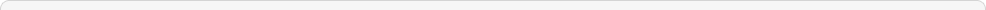Video Tutorials
Getting Started Tutorial and Demo
5 March , 2011
18007 views
Text & ArticlesCreated : Sat , 05 November 2011 21:20   Views : 3212
This value is calculated by first determining the annual Return on Assets for each of the 5 most recent fiscal years and then averaging the resulting values. The Return on Assets is the annual Income After Taxes divided by the Average Total Assets, expressed as a percentage.Created : Sat , 05 November 2011 21:20   Views : 2687
This value is calculated as the Income After Taxes for the most recent fiscal year divided by the Average Total Assets, expressed as a percentage. Average Total Assets is the average of the Total Assets at the beginning and the end of the year.Created : Sat , 05 November 2011 21:20   Views : 2448
This value is calculated by first determining the annual Return on Average Common Equity for each of the 5 most recent fiscal years and then averaging the resulting values. The Return on Common Equity is the annual Income After Taxes divided by the average Common Equity, expressed as a percentage.Created : Sat , 05 November 2011 21:20   Views : 2393
This value is calculated as the Income Available to Common Stockholders for the most recent fiscal year divided by the Average Common Equity and is expressed as a percentage. Average Common Equity is the average of the Common Equity at the beginning and the end of the year.Created : Sat , 05 November 2011 21:20   Views : 2725
This value is calculated by first determining the annual Return on Investment for each of the 5 most recent fiscal years and then averaging the resulting values. The Return on Investment is the annual Income After Taxes divided by the average Total Long Term Debt, Other Long Term Liabilities and Shareholders Equity, and expressed as a percentage.Created : Sat , 05 November 2011 21:20   Views : 2600
This value is the annual Income After Taxes divided by the average Total Long Term Debt, Other Long Term Liabilities, and Shareholders Equity, expressed as a percentage.Created : Sat , 05 November 2011 21:20   Views : 2716
This is the total Selling, General, and Administrative Expenses divided by Total Revenue and is expressed as a percentage for the most recent annual period.Created : Sat , 05 November 2011 21:20   Views : 2409
This is the sum of all short and long term asset categories reported for the most recent fiscal year.Created : Sat , 05 November 2011 21:20   Views : 2490Created : Sat , 05 November 2011 21:20   Views : 2400
This is the annual Book Value for the most recent fiscal year minus Goodwill and Intangible Assets for the same period.Created : Sat , 05 November 2011 21:20   Views : 2430
This is the annual Tangible Book Value divided by the Shares Outstanding at the end of the most recent fiscal year. Tangible Book Value is the Book Value minus Goodwill and Intangible Assets for the same period.Created : Sat , 05 November 2011 21:20   Views : 2509Created : Sat , 05 November 2011 21:20   Views : 2471
This value is calculated by first determining the Effective Tax Rate for the 5 most recent fiscal years and averaging the values. The Effective Tax Rate is defined as Income Taxes (Credit) divided by Income Before Taxes and expressed as a percentage. If the Income Tax is a credit, the result is a Not Meaningful (NM).Created : Sat , 05 November 2011 21:20   Views : 2525
This value is the Income Taxes (Credit) for the most recent year divided by the most recent year's Income Before Taxes and expressed as a percentage. If the Income Tax is a credit, the result is a NM.Created : Sat , 05 November 2011 21:20   Views : 2456
The Effective Tax Rate is the Income Taxes (Credit) for the prior year divided by the Income Before Taxes for the 1st historical fiscal year and expressed as a percentage. If the Income Tax is a credit, the result is a Not Meaningful (NM).Created : Sat , 05 November 2011 21:20   Views : 2613
This is the compound annual growth rate of Current Assets over the last 10 years. NOTE: If the value for either the most recent year or the oldest year is zero or negative, the growth rate cannot be calculated, a 'NA' (Not Available) code will be used.Created : Sat , 05 November 2011 21:20   Views : 2696
This is the compound annual growth rate of Current Assets over the last 5 years. NOTE: If the value for either the most recent year or the oldest year is zero or negative, the growth rate cannot be calculated, a 'NA' (Not Available) code will be used.Created : Sat , 05 November 2011 21:20   Views : 2448
This is the sum of all current and long term liabilities reported for the most recent fiscal year.Created : Sat , 05 November 2011 21:20   Views : 2480
This is an Annual Trend Momentum of Total Assets over 10 years. Trend Momentum is derived for the most recent period by subtracting the log linear Estimated index value (in index value units) from the Actual index value and divide by the Estimated index value to derive a % deviation. Subtract from the most recent period % deviation the % deviation of the prior period. Express this difference in % deviation as a percentage. Required 11 latest fiscal year intervals.Created : Sat , 05 November 2011 21:20   Views : 2380
This is an Annual Trend Growth of Total Assets over 10 years. Trend Growth is the Average Annual incremental log linear slope expressed as a percentage. Required 11 latest fiscal year intervals.